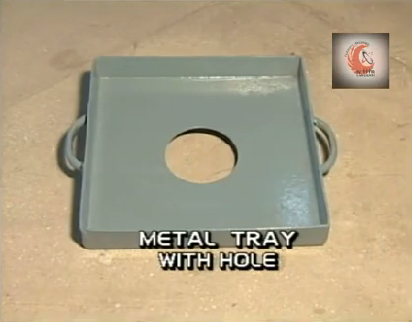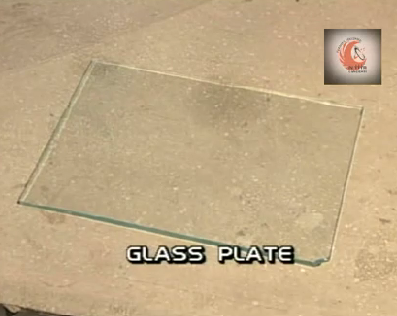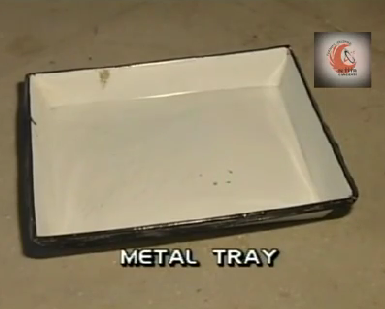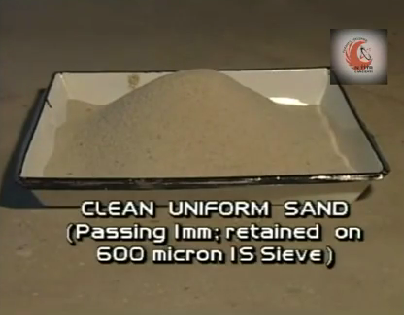# Standard Test Method for Density and Unit Weight of Soil by Sand Replacement Method

Sand replacement test method is used to determine the field density or in-place density of earth embankments, road fills, sub-grade, sub-base or any of compacted material. This method serves as base upon which one can accept the density of a compacted material to a specified magnitude or to a percentage of maximum unit dry density determined as proctor.

As we know that moisture content of the soil vary from time to time and hence the field density also, so we are required to report the test result in terms of dry density. In order to determine the dry density we must have to examine the moisture content in the soil by using general method.
Moisture content (%) = m = ((wt. of wet soil – wt of dry soil) / wt of dry soil)  x 100
Dry density = (bulk density ) / (1 + w)

## Apparatus Required

• Sand pouring cylinder
• Calibrating
• Metal tray
• Excavating tool
• Balance
• Glass plate
• Metal tray
• Clean uniform sand (1mm pass 600 mirco retain)
• Water content determination apparatus## Theory

The dry density of the sample obtained as a result is divided by the proctor test result i.e. the maximum dry obtained of the sample that can be obtained in the laboratory by using standard AASHTO compaction test or Modified AASHTO compaction test and the result is reported as percentage.

The acceptance criteria for these percentages depend on the specification requirements and generally following rules is followed;

No less than 98% within 150 mm below formation level
No less than 95% between 150 mm and 1200 mm below formation
No less than 90% beyond 1200 mm below formation level

## Background

As we know density means weight per unit volume or in other words how much mass is being enclosed in a specific quantum of volume. We can easily determine the mass of soil by using the physical balance or digital balance, but the problem lies in finding the volume of the hole dug.
This problem is solved with the help of a calibrated sand whose unit weight or density is already being determined and thus if we could determine how much weight of calibrated sand is going to rest in the dug hole we can find the volume of the hole by using following formula;
Volume of dug hole = weight of soil in hole dug  / unit weight of calibrated soil

## Procedure

The standard procedure of this test is being divided in two parts in first part we will find the unit weight of the standard soil by calibration process described  as follows;

## Calibration

1. Determine the internal volume of the calibrating container by using the dimensions as follows;
V = πd2h/4
2. Now fill the sand pouring cylinder with the sand to be calibrated within about 10 mm of its top left vacant and then determine the mass of the sand pouring cylinder along with sand and note it as w1.

3. Now place the sand pouring cylinder on top of calibrating cylinder of known volume and open the shutter to allow the sand to fall in to the cylinder after no more sand is falling close the shutter and determine the mass of the calibrating cylinder filled with sand and note it as W2

4. Now as we also have the weight of the sand in the conical portion of the sand pouring cylinder, we must subtract the weight of sand that can accumulate within that conical portion. For that take a flat glass plate and place the sand pouring cylinder. Open the shutter till no more sand falls and determine the mass of sand in the conical portion and note it as W3.

5. Now the weight of the sand in the calibrating cylinder is determined as
Wa = W1 – W2 – W3

6. The bulk density of the sand is determined by dividing the mass of sand in the calibrating cylinder with the volume of the calibrating cylinder.

## Determine the Dry density of the soil under sample

1. Prepare the area of the embankment subject to test, level the top of the soil using the scrapper tool.

2. Place the metal tray on the flat surface, if required insert the nails into the small holes of the metal tray.

3. Trace the circular hole of the tray on the ground and excavate the soil carefully without loosing any of
the soil fragment. Dig a hole of approximately 150 mm in the ground.

4. Collect all the excavated material in a metal container and clear the hole using a brush.

5. Determine the mass of this soil as weight of wet soil from hole Ww.

6. Fill the sand pouring cylinder with the calibrated sand and determine its mass as W1.

7. Place the cylinder directly over the excavated hole. Allow the sand to run out the cylinder by opening the shutter. Close the shutter when the hole is completely filled and no further movement of sand is observed.

8. Now weigh the remaining sand in the sand pouring cylinder and note it as w4.

9. Take a sample of the excavated soil in an air tight sampler for the determination of the water content or moisture content.

10. Volume of the hole is determined by using the unit weight of the calibrated sand already known;

## Observations and Calculations

Volume of calibrating container = V (cm3) = 1000 cm3
Weight of cylinder + sand (before pouring) , W1(g) = 7476 g
Mean weight of cylinder + sand (after pouring), W2 (g) = 5610 g
Mean weight of sand in cone (of pouring cylinder), W3 (g) = 436 g
Weight of sand to fill calibrating container Wa = w1 – w2 – w3 = 1430 g
Bulk density of sand = Gamma b = Wa / v (g/cm3) = 1.43

## Dry Density

Weight of calibrated sand in hole wb = W1 – W4 – W2
Volume of hole = Vh = Wb  / Unit weight of sand
Dry density of soil = ww / vh
% compaction = (dry density / proctor density )  x 100

1.2.